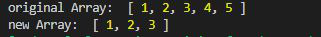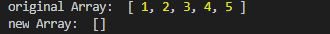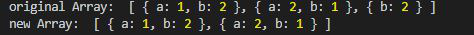GeeksforGeeks App
Open AppBrowser
Continue

# Lodash _.dropRight() Function

Lodash is a JavaScript library that works on the top of underscore.js. Lodash helps in working with arrays, strings, objects, numbers, etc.
The _.dropRight() function is used to delete the elements from the right of the array i.e from the (n-1)th element.

Syntax:

`_.dropRight(array, n)`

Parameter:

• array: It is the original array from which elements are to be deleted.
• n: Here n is the number of elements that are to be deleted from the array. By default, it is set to one.

Return Value: It returns the array.

Note: Install the lodash module by using command `npm install lodash` before using the code given below.

Example 1: When n is less than the size of an array.

 `// Requiring the lodash library``const _ = require(``"lodash"``);`` ` `// Original array``let array1 = [1, 2, 3, 4, 5]`` ` `// Using _.dropRight() function``let newArray = lodash.dropRight(array1, 2);`` ` `// Original Array``console.log(``"original Array: "``, array1)`` ` `// Printing the newArray``console.log(``"new Array: "``, newArray)`

Output:Example 2: When n is greater than the size of the array.

 `// Requiring the lodash library``const _ = require(``"lodash"``);`` ` `// Original array``let array1 = [1, 2, 3, 4, 5]`` ` `// Using _.dropRight() function``let newArray = lodash.dropRight(array1, 10);`` ` `// Original Array``console.log(``"original Array: "``, array1)`` ` `// Printing the newArray``console.log(``"new Array: "``, newArray)`

Output:Example 3: When an array of objects is given and n is not given.

 `// Requiring the lodash library``const _ = require(``"lodash"``);`` ` `// Original array``let array1 = [``    ``{ ``"a"``: 1, ``"b"``: 2 }, ``    ``{ ``"a"``: 2, ``"b"``: 1 }, ``    ``{ ``"b"``: 2 }``]`` ` `// Using _.dropRight() function``let newArray = lodash.dropRight(array1);`` ` `// Original Array``console.log(``"original Array: "``, array1)`` ` `// Printing the newArray``console.log(``"new Array: "``, newArray)`

Output:My Personal Notes arrow_drop_up
Related Tutorials